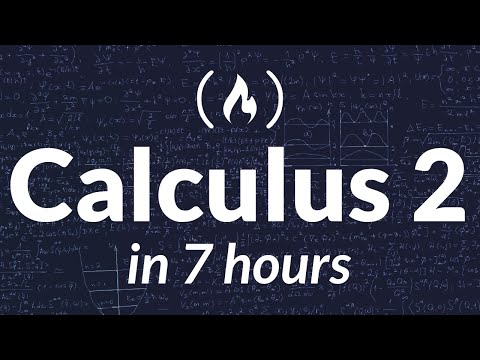Learn Calculus 2 in this full college course.

This course was created by Dr. Linda Green, a lecturer at the University of North Carolina at Chapel Hill. Check out her YouTube channel: https://www.youtube.com/channel/UCkyLJh6hQS1TlhUZxOMjTFw

⭐️ Prerequisites ⭐️

⭐️ Lecture Notes ⭐️
🔗 Calculus 2 Notes: http://lindagreen.web.unc.edu/files/2020/08/courseNotes_m232_2018F.pdf

⭐️ Course Contents ⭐️
⌨️ (0:00:00) Area Between Curves
⌨️ (0:11:11) Volumes of Solids of Revolution
⌨️ (0:21:33) Volumes Using Cross-Sections
⌨️ (0:31:34) Arclength
⌨️ (0:39:50) Work as an Integral
⌨️ (0:51:30) Average Value of a Function
⌨️ (0:59:05) Proof of the Mean Value Theorem for Integrals
⌨️ (1:04:55) Integration by Parts
⌨️ (1:13:03) Trig Identities
⌨️ (1:25:01) Proof of the Angle Sum Formulas
⌨️ (1:29:37) Integrals Involving Odd Powers of Sine and Cosine
⌨️ (1:36:21) Integrals Involving Even Powers of Sine and Cosine
⌨️ (1:44:59) Special Trig Integrals
⌨️ (1:47:36) Integration Using Trig Substitution
⌨️ (1:56:21) Integrals of Rational Functions
⌨️ (2:03:59) Improper Integrals – Type 1
⌨️ (2:10:13) Improper Integrals – Type 2
⌨️ (2:14:20) The Comparison Theorem for Integrals
⌨️ (2:19:43) Sequences – Definitions and Notation
⌨️ (2:31:05) Series Definitions
⌨️ (2:39:41) Sequences – More Definitions
⌨️ (2:46:06) Monotonic and Bounded Sequences Extra
⌨️ (2:49:46) L’Hospital’s Rule
⌨️ (2:57:02) L’Hospital’s Rule on Other Indeterminate Forms
⌨️ (3:06:48) Convergence of Sequences
⌨️ (3:30:38) Geometric Series
⌨️ (3:43:32) The Integral Test
⌨️ (3:56:48) Comparison Test for Series
⌨️ (4:03:29) The Limit Comparison Test
⌨️ (4:09:24) Proof of the Limit Comparison Test
⌨️ (4:16:00) Absolute Convergence
⌨️ (4:25:03) The Ratio Test
⌨️ (4:30:22) Proof of the Ratio Test
⌨️ (4:39:34) Series Convergence Test Strategy
⌨️ (4:50:26) Taylor Series Introduction
⌨️ (5:02:28) Power Series
⌨️ (5:05:42) Convergence of Power Series
⌨️ (5:21:07) Power Series Interval of Convergence Example
⌨️ (5:30:46) Proofs of Facts about Convergence of Power Series
⌨️ (5:36:19) Power Series as Functions
⌨️ (5:43:36) Representing Functions with Power Series
⌨️ (5:52:07) Using Taylor Series to find Sums of Series
⌨️ (6:02:18) Taylor Series Theory and Remainder
⌨️ (6:15:38) Parametric Equations
⌨️ (6:27:06) Slopes of Parametric Curves
⌨️ (6:32:47) Area under a Parametric Curve
⌨️ (6:38:07) Arclength of Parametric Curves
⌨️ (6:45:20) Polar Coordinates